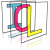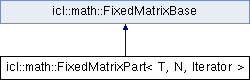Image Component Library (ICL)
icl::math::FixedMatrixPart< T, N, Iterator > Class Template Reference

Utility struct for FixedMatrix sub-parts. More...

`#include <FixedMatrix.h>`

Inheritance diagram for icl::math::FixedMatrixPart< T, N, Iterator >:## Public Member Functions

FixedMatrixPart (Iterator begin, Iterator end)
Creates a new FixedMatrixPart instance with given Iterator Pair. More...

FixedMatrixPartoperator= (const T &value)
Assignment with a new value (all data in range will be assigned with that value) More...

FixedMatrixPartoperator= (const FixedMatrixPart &other)
Assignment with another (identical) instance of FixedMatrixPart. More...

template<class OtherIterator , class OtherT >
FixedMatrixPartoperator= (const FixedMatrixPart< OtherT, N, OtherIterator > &other)
Assignment with another (compatible) instance of FixedMatrixPart. More...

template<unsigned int COLS>
FixedMatrixPartoperator= (const FixedMatrix< T, COLS, N/COLS > &m)
Assignment with a compatible FixedMatrix instance (FixedMatrix DIM must be euqal to Part-size) More...

template<class T2 , unsigned int COLS>
FixedMatrixPartoperator= (const FixedMatrix< T2, COLS, N/COLS > &m)
Assignment with a FixedMatrix instance (FixedMatrix DIM must be euqal to Part-size) More...

## Public Attributes

Iterator begin
Start iterator. More...

Iterator end
End iterator. More...

## Additional Inherited MembersStatic Public Member Functions inherited from icl::math::FixedMatrixBase
template<class SrcIterator , class DstIterator , unsigned int N>
static void optimized_copy (SrcIterator srcBegin, SrcIterator srcEnd, DstIterator dstBegin)
Optimized copy function template (for N>30 using std::copy, otherwise a simple loop is used) More...

## Detailed Description

### template<class T, unsigned int N, class Iterator> class icl::math::FixedMatrixPart< T, N, Iterator >

Utility struct for FixedMatrix sub-parts.

FixedMatrix member functions row and col return an instance of this utility structure. FixedMatrixPart instances wrap a range of Iterators (begin- and end-Iterator) of template parameter type. Once created, FixedMatrixParts can not be setup with different Iterators. All further Assignments will only copy the ranged data represented by the source and destination iterator pairs.\ To avoid problems with ranges of different size, FixedMatrixPart instances are 'templated' to the range size (template parameter N)

## ◆ FixedMatrixPart()

template<class T, unsigned int N, class Iterator>
 icl::math::FixedMatrixPart< T, N, Iterator >::FixedMatrixPart ( Iterator begin, Iterator end )
inline

Creates a new FixedMatrixPart instance with given Iterator Pair.

## ◆ operator=() [1/5]

template<class T, unsigned int N, class Iterator>
 FixedMatrixPart& icl::math::FixedMatrixPart< T, N, Iterator >::operator= ( const T & value )
inline

Assignment with a new value (all data in range will be assigned with that value)

## ◆ operator=() [2/5]

template<class T, unsigned int N, class Iterator>
 FixedMatrixPart& icl::math::FixedMatrixPart< T, N, Iterator >::operator= ( const FixedMatrixPart< T, N, Iterator > & other )
inline

Assignment with another (identical) instance of FixedMatrixPart.

Internally std::copy is used

## ◆ operator=() [3/5]

template<class T, unsigned int N, class Iterator>
template<class OtherIterator , class OtherT >
 FixedMatrixPart& icl::math::FixedMatrixPart< T, N, Iterator >::operator= ( const FixedMatrixPart< OtherT, N, OtherIterator > & other )
inline

Assignment with another (compatible) instance of FixedMatrixPart.

For compatibility. Iterator type and destination type may differ but RangeSize must be equal

## ◆ operator=() [4/5]

template<class T, unsigned int N, class Iterator>
template<unsigned int COLS>
 FixedMatrixPart& icl::math::FixedMatrixPart< T, N, Iterator >::operator= ( const FixedMatrix< T, COLS, N/COLS > & m )

Assignment with a compatible FixedMatrix instance (FixedMatrix DIM must be euqal to Part-size)

DIM equality is forced by Argument template parameters <...,COLS,N/COLS>

## ◆ operator=() [5/5]

template<class T, unsigned int N, class Iterator>
template<class T2 , unsigned int COLS>
 FixedMatrixPart& icl::math::FixedMatrixPart< T, N, Iterator >::operator= ( const FixedMatrix< T2, COLS, N/COLS > & m )

Assignment with a FixedMatrix instance (FixedMatrix DIM must be euqal to Part-size)

DIM equality is forced by Argument template parameters <...,COLS,N/COLS>

## ◆ begin

template<class T, unsigned int N, class Iterator>
 Iterator icl::math::FixedMatrixPart< T, N, Iterator >::begin

Start iterator.

## ◆ end

template<class T, unsigned int N, class Iterator>
 Iterator icl::math::FixedMatrixPart< T, N, Iterator >::end

End iterator.

The documentation for this class was generated from the following file: